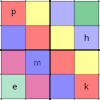# Your Post-16 Solutions### Overturning Fracsum

##### Age 16 to 18Challenge Level

Can you solve the system of equations to find the values of x, y and z?### Always Two

##### Age 14 to 18Challenge Level

Find all the triples of numbers a, b, c such that each one of them plus the product of the other two is always 2.### Symmetricality

##### Age 14 to 18Challenge Level

Five equations and five unknowns. Is there an easy way to find the unknown values?### Simultaneous Equations Sudoku

##### Age 11 to 18Challenge Level

Solve the equations to identify the clue numbers in this Sudoku problem.### Mind Your Ps and Qs

##### Age 16 to 18Challenge Level

Sort these mathematical propositions into a series of 8 correct statements.### Agile Algebra

##### Age 16 to 18Challenge Level

Observe symmetries and engage the power of substitution to solve complicated equations.### Mega Quadratic Equations

##### Age 16 to 18Challenge Level

What do you get when you raise a quadratic to the power of a quadratic?